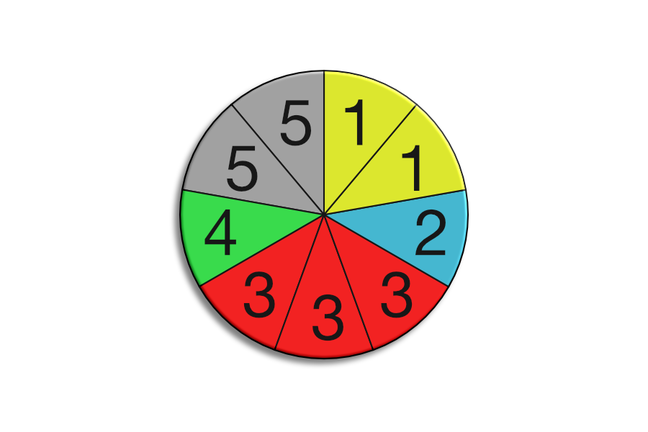## Want to keep learning?

This content is taken from the Cambridge University Press's online course, Teaching Probability. Join the course to learn more.
3.3

## Cambridge University PressSpinner for question 2.

# Using the probability scale

In this step we provide three examples of examination-type questions based on the topics that we looked at in the previous step.

All of this week’s sample questions are intended to be useful for novice and experienced teachers alike.

• In the previous step, we provided a brief review of the material on which these questions are based. You may feel that you need more guidance on the subject matter covered. With all of the questions that we are working on this week, take your time, and feel free to look up methods or solutions elsewhere - this is not a test!
• On the other hand, you may find some or all of the questions we present this week quite easy. That’s fine as well – we want you to think about the difficulties that your students may have with these questions, and how you would teach the material involved.

The questions are below. Work through them on paper, and use the comments to share your solutions and reflections. In the next step we will provide answers, and you will have an opportunity to discuss how you would approach these topics in the classroom.

### Question 1

Consider the following list of numbers.

7, 12, 15, 23, 32, 33, 34, 50

One of these numbers is picked at random. What is the probability that the picked number is:

a odd?

b greater than 6?

c a prime number?

Mark each of these probabilities on a probability scale.

### Question 2

Consider the spinner shown in the diagram (above).

Put a number in each of the spaces to make these statements true.

• There is a probability of $$\frac{1}{3}$$ that the spinner will land on _____ .

• There is a probability of 0 that the spinner will land on _____ .

• The probability of landing on _____ is twice the probability of landing on _____ .

### Question 3

A bag of 50 sweets contains a mixture of red and blue sweets. If I take a sweet at random, the probability of getting a red one is 0.4.

How many blue sweets are in the bag?

### Question 4

Amelia takes a fair coin and flips it 4 times. It comes up: Heads, Tails, Tails, Tails

What is the probability that the next time the coin is flipped it will come up Tails?